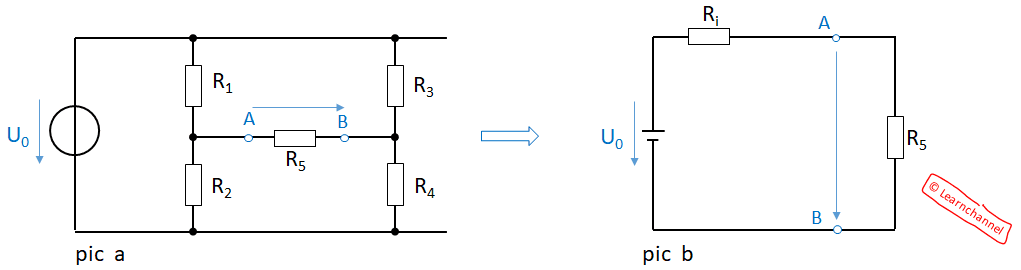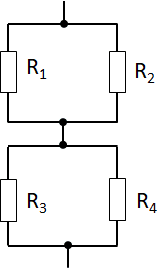# Bridge-Circuit with load

## Calculating a Bridge-Circuit with load

In a loaded bridge circuit, a current between points A and B must be considered. One way to calculate this circuit is to consider the resistor R5 as a load resistor, which is connected to a voltage source with internal resistance Ri:Calculating a Bridge Circuit with load

Specific example with numbers:

U = U0 = 12 V
R1 = 400Ω, R2 = 800Ω, R3 = 600Ω, R4 = 300Ω, R5 = 500Ω

Step 1: Calculating UAB

U2 = R2 / (R1+R2) = 800Ω / 1200Ω  * 12V = 8V
U4 = R4 / (R3+R4) = 300Ω / 900Ω  * 12V = 4V

=> UAB = 8 V - 4 V = 4 V Δ U0, when no current flows, i.e. R5 is not connected!Step 2: Calculation Ri

If we consider the voltage source from figure a to be ideal, it has no internal resistance and can be short-circuited.

Rtotal = Ri = R1 || R2 + R3 || R4 = 466.7Ω

Step 3: Calculation terminal voltage

UAB / R5  = U0 / (R5 + Ri)

=>   UAB =  2,07 V